# 2nd PUC Maths Question Bank Chapter 12 Linear programming

Students can Download 2nd PUC Maths Chapter 12 Linear programming Questions and Answers, Notes Pdf, 2nd PUC Maths Question Bank with Answers helps you to revise the complete Karnataka State Board Syllabus and to clear all their doubts, score well in final exams.

## Karnataka 2nd PUC Maths Question Bank Chapter 12 Linear programming

### 2nd PUC Maths Linear programming One Marks Questions and Answers

Question 1.
Define optimal solution in linear programming problem.
Any feasible solution of LPP which maximizes or minimizes the objective function is called an optimal solution.

Question 2.
Define feasible region.
The region containing the set of points satisfying all the constraints of an LPP is called the feasible region.Question 3.
Define the term “objective function” in an L.P.P.
The linear function Z= ax + by where a and b are constants which has to be maximised or minimised is called a linear objective function.

Question 4.
Define the term ‘constraints’ in a LPP.
The linear inequalities or equations or restrictions on the variables of a linear programming problem are called constraints.

Question 5.
Define the optimisation problem.
Optimisation problem is a problem which seeks to maximise or minimise a linear function in two variables x and y subject to certain constraints as determined by a set of linear inequalities is called an optimisation problem.

Question 6.
Define the term Feasible Solution in a LPP.
The points within and on the boundary of the feasible region represent feasible solutions of the constraints.

Question 7.
Define infeasible solution.
Any point outside the feasible region is called an infeasible solution.

Question 8.
Define the term corner point of a feasible region in an LPP.
A corner point of a feasible region is a point in the region which is the intersection of two boundary lines.### 2nd PUC Maths Linear programming Six Marks Questions and Answers

Question 1.
Maximize Z = 3x + 4y, subject to the constraints are x + y ≤ 4, x ≥ 0 and y ≥ 0.
Draw the graph of the line x + y = 4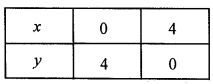Putting (0, 0) in the inequality x + y ≤ 4, we have 0 + 0 ≤ 4 ⇒ 0 ≤ 4 (which is true)
So, the half plane is to wards the origin, Since, x, y ≥ 0
So, the feasible region lies in the first quadrant.
∴ Feasible region is OABO.
The corner points of the feasible region are 0(0, 0), A (4, 0) and B(0, 4). The values of Z at these points are as follows:Therefore, the maximum value of Z is 16 at the point B(0, 4).Question 2.
Maximize Z = – 3x + 4y, subject to constraints are x + 2y ≤ 8, 3x + 2y ≤ 12, x ≥ 0 and y ≥ 0.
Draw the graph of the line x + 2y = 8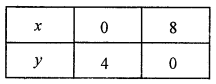Putting (0, 0) in the inequality x + 2y ≤ 8, we have
0 + 0 ≤ 8 ⇒ 0 ≤ 8
(which is true)
So, the half plane is to wards the origin,
Since,
x, y ≥ 0
So the feasible region lies in the first quadrant.
Draw the graph of the line, 3x + 2y = 12Putting (0, 0) in the in equality 3x + 2y ≤ 12, we have
3 × 0 + 2 × 0 ≤ 12 ⇒ 0 ≤ 12
(which is true)
So, the half plane is to wards the origin,
∴ Feasible region is OABCO.
On solving equations x + 2y = 8 and x = 2 and y = 3
∴ Intersection point B, is (2, 3)
The corner points of the feasible region are 0(0, 0), A (4, 0) and B(2, 3) and C(0, 4). The values of Z at these points are as follows: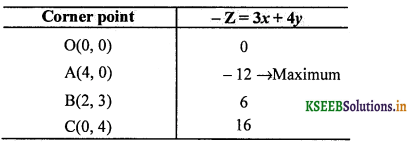Therefore, the maximum value of Z is – 12 at the point A(4, 0).Question 3.
Maximize Z = 5x + 3y, subject to constraints are 3x + 5y ≤ 15, 5x + 2y ≤ 10, x ≥ 0 and y ≥ 0.
Draw the graph of the line, 3x + 5y = 15Putting (0, 0) in the inequality 3x + 5y ≤ 15, we have
3 × 0 + 50 × 0 ≤ 15 ⇒ 0 ≤ 15
(which is true)
So, the half plane is towards the origin.
Since, x, y ≥ 0
So, the feasible region lies in the first quadrant.
Draw the graph of the line, 5x + 2y = 10Putting (0, 0) in the inequality 5x + 2y ≤ 10, we have
5 × 0 + 2 × 0 ≤ 10 ⇒ 0 ≤10
(which is true)
So, the half plane is towards the origin.
On solving given equations 3x + 5y = 15 and 5x + 2y = 10, we get x = $$\frac { 20 }{ 19 }$$, y = $$\frac { 45 }{ 19 }$$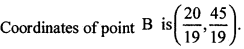∴ Feasible region is OABCO.The values of Z at these points are as follows:Question 4.
Minimize Z = 3x + 5y, subject to constraints are x + 3y ≤ 3, x + y ≤ 2 and x, y ≥ 0.
Draw the graph of the line, x + 3y = 3Putting (0, 0) in the inequality x + 3y ≤ 3, we have
0 + 3 × x ≤ 3 ⇒ 0 ≤ 3
(which is false)
So, the half plane is away from the origin. Since, x, y ≥ 0
So, the feasible region lies in the first quadrant.
Draw the graph of the line, x + y = 2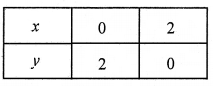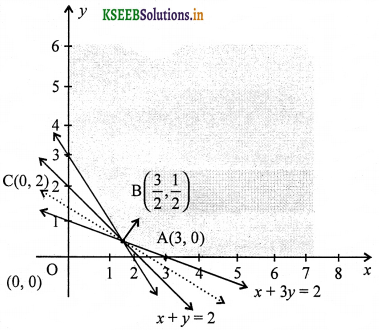Putting (0, 0) in the inequality x + y ≤ 2, we have
0 + 0 ≤ 2 ⇒ 0 ≤ 2
(which is false)
So, the half plane is away from the origin.
It can be seen that the feasible region is unbounded.
On solving given equations x + y = 2 and x + 3y = 3, we get
x = $$\frac { 3 }{ 2 }$$ and y = $$\frac { 1 }{ 2 }$$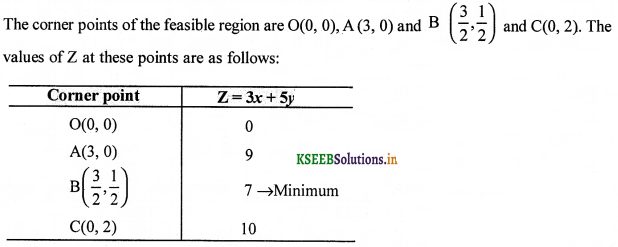As the feasible region is unbounded therefore, 7 may or may not be the minimum value of Z. For this, we draw the graph of the inequality, 3x + 5y < 7 and check whether the resulting half plane has points in common with the feasible region or not.
It can be seen that the feasible region has no common point with 3x + 5y < 7.Question 5.
Minimize Z = 3x + 2y, subject to constraints are x + 2 ≤ 10, 3x + y ≤ 15, and x, y ≥ 0.
Draw the graph of the line, x + 2y= 10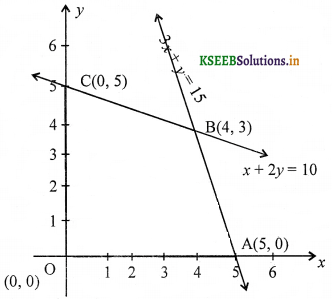Putting (0, 0) in the inequality x + 2y ≤ 10. we have
0 + 2 × 0 ≤ 10 ⇒ 0 ≤ 10
(which is true)
So, the half plane is towards the origin.
Since, x, y ≥ 0
So, the feasible region lies in the first quadrant.
Draw the graph of the line, 3x + y = 15Putting (0, 0) in the inequality 3x + y ≤ 15, we have
3 × 0 + 0 ≤ 15 ⇒ 0 ≤ 15 (which is true).
So, the half plane is towards the origin.
On solving equations x + 2y= 10 and 3x + y = 15, we get x = 4 and y = 3
∴ Intersection point B is (4, 3)
∴ Feasible region is OABCO.
The corner points of the feasible region are 0(0, 0), A (5, 0) and B(4, 3) and C(0, 5). The values of Z at these points are as follows: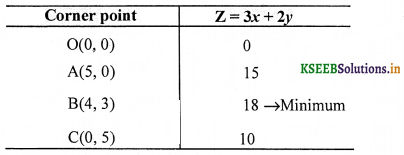Therefore, the maximum value of Z is 18 at the point B(4, 3).Question 6.
Minimize Z = x + 2y, subject to constraints are 2x + y ≥ 3, x + 2y ≥ 6, and x, y ≥ 0. Show that the minimum of Z occurs at more than two points.
Draw the graph of the line, 2x + 2y = 3z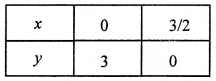Putting (0, 0) in the inequality 2x + y ≥ 3,
we have 2 × 0 + 0 ≥ 3 ⇒ 0 ≥ 3
(which is false)
So, the half plane is away form the origin. Since, x, y ≥ 0
So, the feasible region lies in the first quadrant.
Draw the graph of the line, x + 2y = 6Putting (0, 0) in the inequality x + 2y ≥ 6, we have
0 + 2 × 0 ≥ 6 ⇒ 0 ≥ 6
(which is false)
So, the half plane is to wards the origin.
The intersection point of the lines x + 2y = 6 and 2x + y = 3 is B(0, 3).
The comer points of the feasible region are A (6, 0) and B(0, 3). The values of Z at these points are as follows: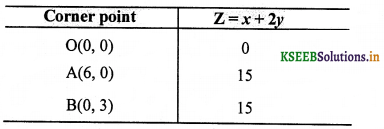It can be seen that the value of Z at points A and B is same. If we take any other point such as (2, 2) on line x + 2y = 6, then Z = 6. Thus, the minimum value of Z occurs for more than 2 points. Therefore, the value of Zis minimum at every point on the line, x + 2y = 6.Question 7.
Maximize and Minimize Z = 5x + 10y, subject to constraints are x + 2y ≤ 120, x + y ≥ 60, x – 2y ≥ 0 and x,y ≥ 0.
Draw the graph of the line, x + 2y = 120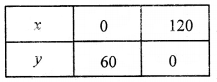Putting (0, 0) in the inequality x + 2y ≤ 120, we have
0 + 2 × 0 ≤ 120 ⇒ 0 ≤ 120
(which is true)
So, the half plane is towards the origin.
Draw the graph of the line, x + y = 60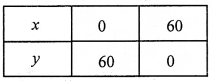Putting (0, 0) in the inequality x + y ≥ 60, we have
0 + 0 ≤ 60 ⇒ 0 ≤ 60
(which is false)
So, the half plane is away from the origin.
Draw the graph of the line x – 2y = 0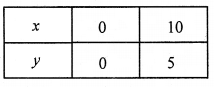Putting (5, 0) in the inequality
x – 2y ≥ 0, we have
5 – 2 × 0 ≥ 0 ⇒ 5 ≥ 0
(which is true)
So, the half plane is towards the X-axis.
Since, x, y ≥ 0
So, the feasible region lies in the first quadrant.
∴ Feasible region is ABCDA.
On solving equations x – 2y = 0 and x + y = 60, we get D (40, 30).
and on solving equations x – 2y = 0 and x + 2y = 120, we get C(60, 30).
The corner points of the feasible region are A(60, 0), B(120, 0), C(60, 30) and D(40,20). The values of Z at these points are as follows:The minimum value of Z is 300 at (60, 0) and the maximum value of Z is 600 at all the points on the line segment joining the points (120, 0) and (60, 30).Question 8.
Minimise and Maximise Z = x + 2y, subject to constraints are x + 2y ≥ 100, 2x – y ≤ 0, 2x + y ≤ 200 and x, y ≥ 0.
Draw the graph of the line, x + 2y = 100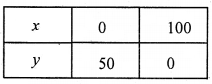Putting (0, 0) in the inequality x + 2y ≥100, we have
0 + 2 × 0 ≥ 100 ⇒ 0 ≥ 100
(which is false)
So, the half plane is away the origin.
Draw the graph of the line, 2x – y = 0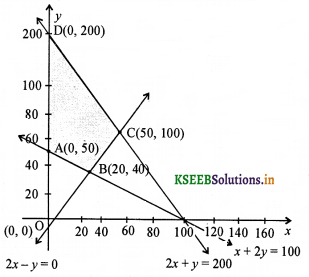Putting (5, 0) in the inequality 2x – y ≤ 0, have
2 × 5 – 0 ≤ 0 ⇒ 10 ≤ 0
(which is false)
So, the half plane is towaids Y-axis.
Draw the graph of the line 2x + y – 200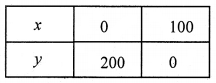Putting (0, 0) in the inequality 2x + y ≤ 200, we have
2 × 0 + 0 ≤ 200 ⇒ 0 ≤ 200
(which is true)
So, the half plane is towards the origin. Since, x, y ≥ 0
So, the feasible region lies in the first quadrant.
On solving equations 2x – y = 0 and x + 2y = 100, we get B(20, 40)
and on solving the equations 2x – y = 0 and 2x + y = 200, we get C(50, 100).
∴ Feasible region is ABCDA.
The corner points of the feasible region are A(0, 50), B(20, 40), C(50, 100) and D(0, 200). The values of Z at these points are as follows: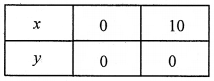Putting (5, 0) in the inequality x – 2y ≥ 0, we have
5 – 2 × 0 ≥ 0 ⇒ 5 ≥ 0
(which is true)
So, the half plane is towards the X-axis. Since, x, y > 0
So, the feasible region lies in the first quadrant.
∴ Feasible region is ABCDA.
On solving equations x – 2y = 0 and x + y – 60, we get D (40, 30).
and on solving equations x – 2y = 0 and x + 2y = 120, we get C(60, 30),
The corner points of the feasible region are A(60, 0), B(120, 0), C(60, 30, 3) and C(40, 20). The values of Z at these points are as follows: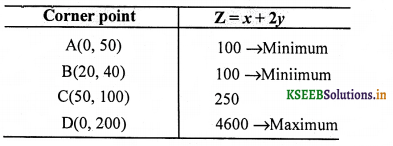The maximum value of Z is 400 at D(0, 200) and the minimum value of Z is 100 at all the points on the line segment joining the points (0, 50) and B(20, 40).Question 9.
Minimize Z = -x + 2y, subject to constraints are x ≥ 3, x + y ≥ 5, x + 2y ≥ 6 and x, y ≥ 0.
Draw the graph of the line, x + y = 5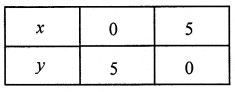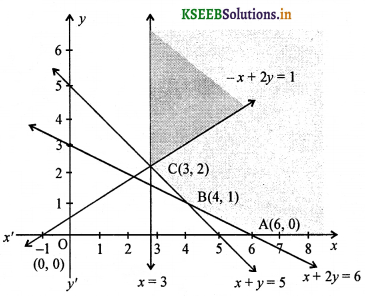Putting (0, 0) in the inequality x + y ≥ 5, we have
0 + 0 ≥ 5 ⇒ 0 ≤ 5
(which is false)
So, the half plane is ay from the origin.
Draw the graph of the line, x + 2y = 6Putting (0, 0) in the inequality x + 2y ≥ 6, we have
0 + 2 × 0 ≥ 6 ⇒ 0 ≥ 6
(which is false)
So, the half plane is away from the origin.
Draw the graph of the line – x + 2y= 1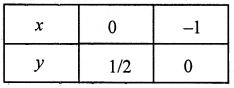Putting (0, 0) in the inequality -x + 2y > 1, we have
-0 + 2 × 0 > 1 ⇒ 0 > 1
(which is false)
So, the half plane is away the origin. Since, x ≥ 3, y ≥ 0
So, the feasible region lies in the first quadrant.
The points of intersection of lines x = 3 and – x + 2y = 1 is C(3, 2) and lines x + 2y = 6 and x + y = 5 is B(4, 1).
It can be seen that the feasible region is unbounded.
The corner points of the feasible region are A(6, 0), B(4, 1) and C(3, 2). The values of Z at these points are as follows: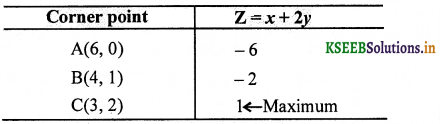As the feasible region is unbounded therefore, Z = 1 may or may not be the maximum value. For this, we graph the inequality, – x + 2y > 1 and check whether the resulting half plane has points in common with the feasible region or not.
The resulting feasible region has points in common with the feasible region. Therefore, Z = 1 is not the maximum value.
Hence, Z has no maximum value.Question 10.
Maximize Z = x + y, subject to constrainsts are x – y ≤ -1,-x + y ≤ 0 and x,y ≥ 0.
Draw the graph of the line x – y = – 1Putting (0, 0) in the inequality x – y ≤ -1, we have
0 – 0 ≤ – 1 ⇒ 0 ≤ – 1
(which is false)
So, the half plane is away from the origin.
Draw the graph of the line – x + y = 0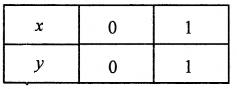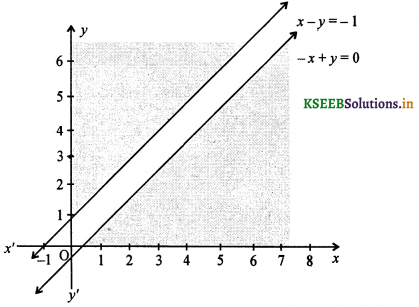Putting (2, 0) in the inequality
-x + y ≤ 0, we have
– 2 + 0 ≤ 0 ⇒ -2 ≤ 0
(which is true)
So, the half plane is towards the X-axis.
Since, x,y ≥ 0
So, the feasible region lies in the first quadrant.
From the above graph, it is clearly shown that there is no common region. Hence, there is no feasible region and thus Z has no maximum value.Question 11.
Reshma wishes to mix two types of food P and Q in such a way that the vitamin contents of the mixture contain atleast 8 units of vitamin A and 11 units of vitamin B. Food P costs ₹ 60 per kg and food Q costs ₹ 80 per kg. Food P contains 3 units per kg of vitamin A and 5 units per kg of vitamin B while food Q contains 4 units per kg of vitamin A and 2 units per kg of vitamin B. Determine the minimum cost of the mixture.
Let Reshma mxes x kg of food P and y kg of food Q. Construct the following table.The mixture must contain atleast 8 units of vitamin A and 11 units of vitamin B. Total cost Z
of purchasing food is Z = 60x + 80y
The mathematical formulation of the given problem is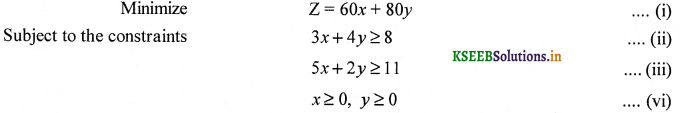Draw the graph of the line 3x + 4y = 8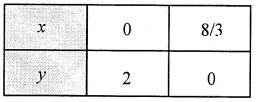Putting (0, 0) in the inequality 3x + 4y ≥ 8, we have
3 × 0 + 4 × 0≥ 8
⇒ 0 ≥ 8
(which is false)
So, the half plane is away from the quadrant.
Draw the graph of the line 5x + 2y = 11Putting (0, 0) in the inequality 5x + 2y ≥ 11, we have
5 × 0 + 2 × 0 ≥11
⇒ 0 ≥ 11
(which is false)
So, the half plane is away from the origin.
It can be seen that the feasible region is unounded.
On solving equations 3x + 4y – 8 and 5x + 2y = 11,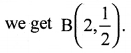The corner points of the feasible region are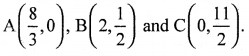The values of Z at these points are as follows: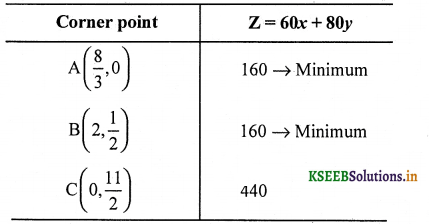As the feasible region is unbounded, therefore 160 may or may not be the minimum value of Z. For this, we graph the inequality 60x + 80y < 160 or 3x + 4y < 8 and hceck whether the resulting half plane has points in common with the feasible region or not. It can be seen that the feasible region has no common point with 3x + 4y < 8 therefore,Theminimum cost of the mixture will be ₹ 160 at the line segment joining the points A$$\left(\frac{8}{3}, 0\right)$$ and B$$\left(2, \frac{1}{2}\right)$$.Question 12.
One kind of cake requires 200 g of flour and 25 g of fat and another kind of cake requires 100 g of flour and 50 g of fat. Find the maximum number of cakes which can be made from 5 kg of flour and 1 kg of fat assuming that there is no shortage of the other ingredients used in making the cakes.
Let x be number of cakes of one kind and y be the number of cakes of other kind. Construct the following table:Draw the graph of the line 2x + y = 50Putting (0, 0) in the inequality
2x + y ≤ 50, we have
2 × 0 + 0 ≤ 50, ⇒ 0 ≤ 50
(which is true)
So, the half plane is towards the orgin.
Draw the graph of the line x + 2y = 40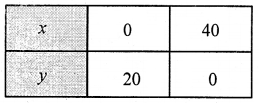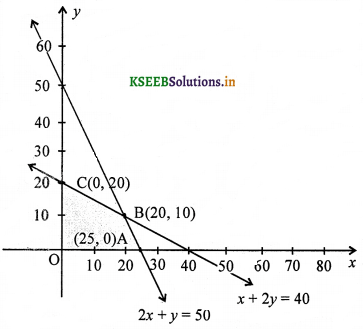Putting (0, 0) in the inequality
2x + y ≤ 40, we have
0 + 2 × 0 ≤ 40
⇒ 0 ≤ 40
(which is true)
So, the half plane is towards the origin. Since, x,y ≥ 0
So, the feasible region lies in the first quadrant.
On solving equations 2x + y = 50 and x + 2y = 40, we get B (20, 10)
∴ Feasible region is OABCO.
The corner points of the feasible region are 0 (0, 0), A(25, 0), B(20, 10) and C(0, 20). The values of Z at these points are as follows:’Thus, the maximum number of cakes that can be made is 30 i.e., 20 of one kind and 10 of the other kind.Question 13.
A factory makes tennis rackets and cricket bats. A tennis racket takes 1.5 h of machine time and 3 h of craftman’s time in its making while a cricket bat takes 3 h of machine time and 1 h of craftman’s time. In a day, the factory has the availability of not more than 42 h of machine time and 24 h of craftman’s time.
(a) What number of rackets and bats must be made, if the factory is to work at full capacity?
(b) If the profits on rackets and on bats is ₹ 20 and ₹ 10 respectively, find the maximum profit of the factory when it works at full capacity.
Let the number of rackets and the number of cricket bats to be made in a day be x and y respectively.
Construct the following table:The machine time is not available for more than 42 h. ∴ 1.5x + 3y ≤ 42
The craftman’s time is not available for more than 24 h. ∴ 3x + y ≤ 24
The profit on rackets is ₹ 20 and on bats is ₹ 10.Draw the graph of the line 1.5x + 3y = 42Putting (0, 0) in the inequality
1.5x + 3y ≤ 42,we have
1.5 × 0 + 3 × 0 ≤ 42 ⇒ 0 ≤ 42
(which is true)
So, the half plane is towards the origin.
Since, x,y ≥ 0
So, the feasible region lies in the first quadrant.
Draw the graph of the line 3x + y = 24Putting (0, 0) in the inequality 3x + y ≤ 24, we have
3 × 0 + 0 ≤ 24 ⇒ 0 ≤ 24
(which is true)
So, the half plane is towards the origin.
On solving equations 1.5x + 3y = 42 and 3x + y = 24, we get B(4, 12).
∴ Feasible region is OABCO.
The corener points of the feasible region are O (0, 0) A(8, 0), B(4, 12) and C(0, 14). The values of Z at these pojpts are as follows: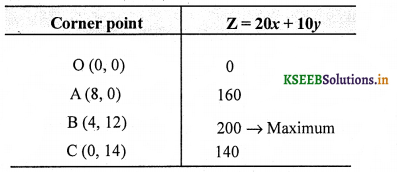Thus, the maximum profit of the factory when it works to its full capacity is ₹ 200.Question 14.
A manufacturer produces nuts and bolts. It takes 1 hr of work on machine A and 3 hr on machine B to produce a package of nuts. It bolts. He earns a profit of ₹ 17.50 per package on nuts and ₹ 7.00 per package on bolts. How many package of each should be produced each most 12 h a day?
Let the manufacturer produces x nuts and y bolts.
We construct the following table: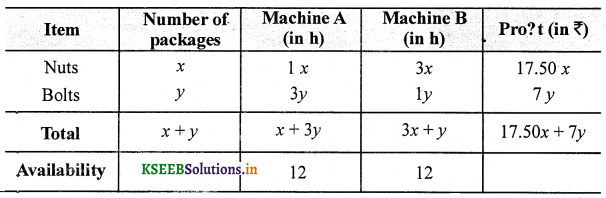∴ Total profit, Z = 17.5x + 7y i.e,, maximize Z = 17.5x + 7y …. (i)
Subject to constraints
x + 3y ≤ 12 …. (ii)
3x + 4y ≤ 12 …. (iii)
x ≥ 0, y ≥0
Draw the graph of the lines x + 3y = 12Putting (0, 0) in the inequality x + 3y ≤ 12, we have
0 + 3 × 0 ≤ 12
⇒ 0 ≤ 12
(which is true)
So, the half plane is towards from the origin.
Since, x,y ≤ 0
So, the feasible region lies in first quadrant.
Draw the graph of the line 3x + y = 12Putting (0, 0) in the inequality
3x + y ≤ 12, we have
3 × 0 + 0 ≤12,
⇒ 0 ≤ 12
(which is true)
So, the half plane is towards from the origin.
On solving equations x + 3y = 12 and 3x + y – 12, we get B(3, 3).
∴ Feasible region is OABCO.
The corner points.of the feasible region are 0(0, 0) A(4, 0), B(3,3) and C(0, 4). The values of Z at these (0, 0) points are as follows: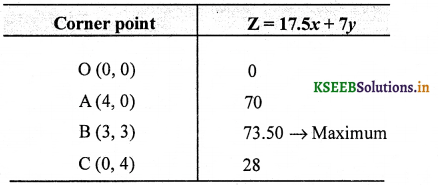The maximum value of Z is ₹ 73.50 at (3, 3).
Thus, 3 packages of nuts and 3 packages of bolts should be produced each day to get te maximum profit ₹ 73.50.Question 15.
A factory manufactures two types of screws A and B. Each type of screw requires the use of two machines, an automatic and a hand operated. It takes 4 min on the automatic and 6 min on hand operated machines to manufacture a package of screws A, while it takes 6 min on automatic and 3 min on the hand operated machines to manufacture a package of screws B. Each machine is available for at the most 4 h on any day. The manufacturer can sell a package of screws A at a profit of ₹ 7 and screws B at a profit of ₹ 10. Assuming that he can sell all the screws he manufactured, how many packages of each type should the factory owner produce in a day in order to maximize his profit? Determine the maximum profit.
Let the manufacturer produces x package of screws A andy y package of screws B. We construct the following table: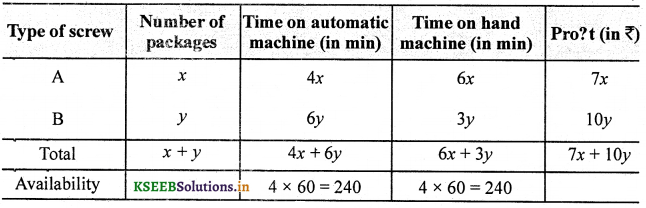The profits on a package of screws A is ₹ 7 and on the package of screws B is ₹ 10.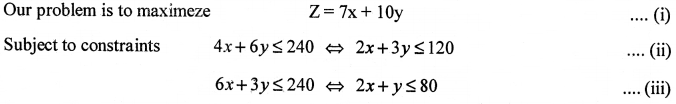Draw the graph of the line 2x + 3y= 120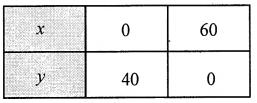Putting (0, 0) in the inequality)
2x + 3y ≤ 120,we have
2 × 0 + 3 × 0 ≤ 120
⇒ 0 ≤ 120
(which is true)
So, the half plane is towards the origin.
Draw the graph of the line 2x + y = 80.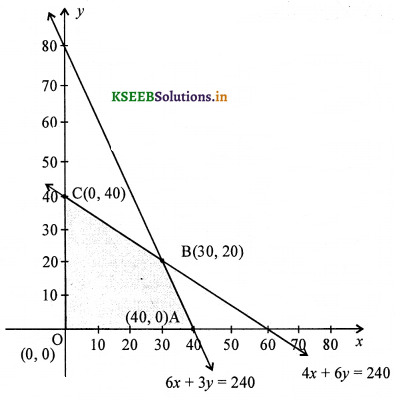Putting (0, 0) in the inequality
2x + y ≤ 80, we have
2 × 0 + 0 ≤ 80 ⇒ o ≤ 80
(which is true)
So, the half plane is towards the origin. Since, x,y ≥ 0
So, the feasible region lies in the first quadrant.
On solving equations
2x + 3y = 120 and 2x + y = 80,
we get B(30, 20).
The corner points of the feasible region are 0(0, 0), A(40, 0), B(30, 20) and C(0, 40).
The values of Z at these points are as follows:
∴ Feasible region is OABCO.The maximum value of Z is ₹ 410 at B(30, 20).
Thus, the factory should produce 30 packages of screws A and 20 packages of screws B to get the maximum profit of ₹ 410.